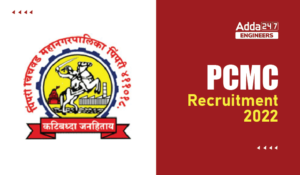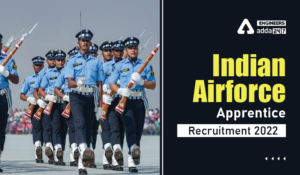Engineering Jobs   »   Mechincal Engineering quizs   »   Mechanical Engineering Quiz

# UPRVUNL’21 ME: Daily Practices Quiz. 7-Sep-2021

Quiz: Mechanical Engineering
Exam: UPRVUNL
Topic: Miscellaneous

Each question carries 1 mark
Negative marking: 1/4 mark
Time: 8 Minutes

Q1. The instrument preferred in the measurement of highly fluctuating velocities in air flows is
(a) Pitot-static tube
(b) Propeller type anemometer
(c) Three cup anemometer
(d) Hot wire anemometer

Q2. For an inclined plane submerged in a liquid, the centre of pressure on one side of the plane will be
(a) above the top edge of the area
(b) vertically below the center of gravity
(c) below the center of gravity
(d) in the same horizontal plane as the center of gravity

Q3. For an irrotational motion
(a) The fluid element does not undergo any shear
(b) The fluid particles do not undergo a circular motion
(c) The circulation around any path is zero
(d) The viscosity may not be zero

Q4. Match List-I (Measuring device) with List-II (Parameters) regarding a body partly submerged in a liquid and select the correct answer using the codes given below the lists:
List-I List-II
A. Anemometer 1. Flow rate
B. Piezometer 2. Velocity
C. Pitot tube 3. Static pressure
D. Orifice 4. Difference between static and stagnation pressure
Codes :
A B C D
(a) 1 3 4 2
(b) 1 2 3 4
(c) 2 3 4 1
(d) 2 4 3 1

Q5. Factor of safety is defined as
(a) Tensile stress/permissible stress
(b) Compressive stress/Ultimate stress
(c) Ultimate stress/Permissible stress
(d) Ultimate stress/Shear stress

Q6. What is the maximum shear stress on the wall of a thin cylinder of diameter (d), thickness (t) and the gauge pressure (p) is?
(a) pd/t
(b) pd/4t
(c) pd/2t
(d) pd/8t

Solution

S1. Ans (d)
Sol. Hot wire anemometer is used for the measurement of highly fluctuating velocities in air. This can be done by measuring the heat loss of the wire which is placed in the fluid stream. The wire is heated by electrical current. The hot wire when placed in the stream of the fluid, in that case, the heat is transferred from wire to fluid, and hence the temperature of wire reduces. The resistance of wire measures the flow rate of the fluid which is the function of heat loss from the wire and change in the resistance of wire.

S2. Ans (c)
Sol. For an inclined plane submerged in a liquid, the center of pressure is below the center of gravity.

S3. Ans.(c)
Sol.
For irrotational motion, the circulation around any path is zero because circulation is the product of vorticity and area. Vorticity is twice of rotation. Hence for an irrotational motion, vorticity is zero and hence circulation is zero.

S4. Ans.(c)
Sol.
Anemometer: It is device for measuring wind speed, and it a common weather station instrument.
Orifice plate: It is a device used for measuring the volumetric flow. It uses the Bernoulli’s principle.
S5. Ans. (c)
Sol. Factor of safety is defined as the ratio of ultimate stress to the working stress (i.e. permissible stress)
FOS=(Ultimate stress)/(Permissible stress)
S6. Ans. (b)
Sol. the state of stress on the wall of a thin cylinder simplifies to a simple two dimensional system with principal stress σ_C and σ_l (by neglecting p)
σ_c (Circumfencial or hoop stress)=pd/2t
σ_l (longitudinal stress)=pd/4t
τ_max=1/2 (σ_C )
=1/2 [pd/2t]=pd/4t

Sharing is caring!

•MP Vyapam Sub Engineer Notification 2022...
•PCMC Recruitment 2022, Check here the de...
•BEL Recruitment 2022, Check here For 13 ...
•Indian Air Force Apprentice Recruitment ...
•BPSC Exam Calendar 2022, Check BPSC Assi...
•SSC Scientific Assistant IMD Recruitment...